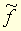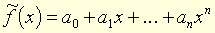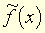# polynomial

﻿
polynomial

polynomial, polynomiale, polynomiaux adjectif Fonction polynomiale associée à un polynôme à une indéterminée, fonction, notée, qui à f = a0 + a1X + … + anXn associe. [est appelé valeur du polynôme f en x.] ● polynomial, polynomiale, polynomiaux (expressions) adjectif Fonction polynomiale associée à un polynôme à une indéterminée, fonction, notée, qui à f = a0 + a1X + … + anXn associe. [est appelé valeur du polynôme f en x.] ● polynomial, polynomiale, polynomiaux (synonymes) adjectif Fonction polynomiale associée à un polynôme à une indéterminée
Synonymes :
- fonction polynôme

Encyclopédie Universelle. 2012.

### Regardez d'autres dictionnaires:

• Polynomial — Pol y*no mi*al, a. 1. Containing many names or terms; multinominal; as, the polynomial theorem. [1913 Webster] 2. Consisting of two or more words; having names consisting of two or more words; as, a polynomial name; polynomial nomenclature. [1913 …   The Collaborative International Dictionary of English

• Polynomial — Pol y*no mi*al, n. [Poly + nomial, as in monomial, binomial: cf. F. polyn[^o]me.] (Alg.) An expression composed of two or more terms, connected by the signs plus or minus; as, a^{2} 2ab + b^{2}. [1913 Webster] …   The Collaborative International Dictionary of English

• polynomial — 1670s (n.), 1704 (adj.), irregularly formed from POLY (Cf. poly ) + stem of BINOMIAL (Cf. binomial) …   Etymology dictionary

• polynomial — [päl΄i nō′mē əl] n. [ POLY + (BI)NOMIAL] an expression or name consisting of more than two terms; specif., a) Algebra a linear combination of products of integral powers of a given set of variables, with constant coefficients (Ex.: x3 + 3x + 2 or …   English World dictionary

• Polynomial — In mathematics, a polynomial (from Greek poly, many and medieval Latin binomium, binomial   , the word has been introduced, in Latin, by Franciscus Vieta) is an expression of finite length constructed from variables (also known as… …   Wikipedia

• polynomial — /pol euh noh mee euhl/, adj. 1. consisting of or characterized by two or more names or terms. n. 2. Algebra. a. (in one variable) an expression consisting of the sum of two or more terms each of which is the product of a constant and a variable… …   Universalium

• polynomial — UK [ˌpɒlɪˈnəʊmɪəl] / US [ˌpɑlɪˈnoʊmɪəl] noun [countable] Word forms polynomial : singular polynomial plural polynomials maths an expression in algebra (= a type of mathematics) that contains two or more terms Derived word: polynomial UK / US… …   English dictionary

• polynomial — I. noun Etymology: poly + nomial (as in binomial) Date: 1674 a mathematical expression of one or more algebraic terms each of which consists of a constant multiplied by one or more variables raised to a nonnegative integral power (as a + bx +… …   New Collegiate Dictionary

• Polynomial — Polynôme Courbe polynomiale cubique Un polynôme, en mathématiques, est la combinaison linéaire des produits de puissances d une ou de plusieurs indéterminées, habituellement notées X, Y, Z… Ces objets sont largement utilisés en pratique, ne… …   Wikipédia en Français

• polynomial — 1. adjective a) Able to be described or limited by a polynomial. b) of a polynomial name or entity 2. noun a) An expression consisting of a sum of a finite number of terms, each term being the product of a constant coefficient and one or more… …   Wiktionary